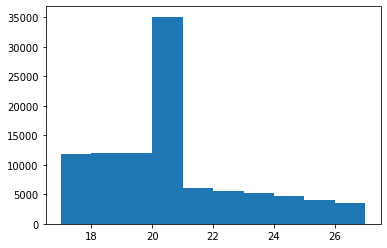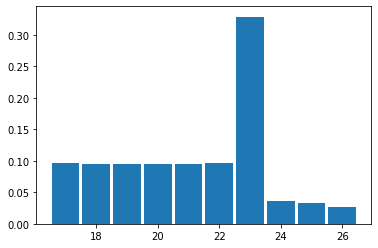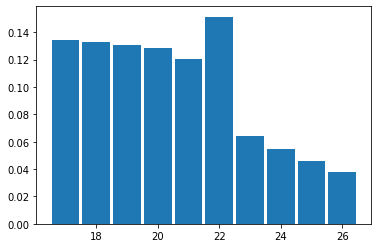# The story of blackjack A processing (python)

I was addicted to the blackjack of Asobi Taizen, so I tried to show the distribution of the total values of the parents in a histogram.

At that time, I stumbled upon the process of A, I dealt with it as follows.

# Conclusion

Instead of treating A as basic 11, Set the "grace" parameter. When A is subtracted, the parameter is incremented by 1. If the parameter is 1 or more when it exceeds 21 Reduce 10 from the total and return

# Blackjack function basics

Let's first consider the case of treating A as just one.

First install the module

``````import numpy as np
import matplotlib.pyplot as plt
import numpy.random as random
import pandas as pd
%matplotlib inline
``````

Card drawing function deal A function that draws cards and returns the result until the total reaches 17. Dealer Normal_deck representing a bunch of cards

``````def deal(x,deck):
x += random.choice(deck)
return x
``````
``````def dealer(x,deck):
while x < 17 :
x = deal(x,deck)
return x
``````
``````normal_deck = [1,2,3,4,5,6,7,8,9,10,10,10,10]*4
``````

Now with dealer (total of parents, card to use) You will get the total value of the final parent card. Let's try turning it about 100,000 times and display the data in a histogram. This is an example of drawing a card from a state where the total value of the parents is 10.

``````ln =[]
for i in range(100000):
ln.append(dealer(10,normal_deck))

bins= [17,18,19,20,21,22,23,24,25,26,27]
plt.hist(ln, bins=bins)
``````

resultThe vertical axis can be a relative frequency or various.

# Version dealing with A

When A comes out, it is calculated as 11. However, when it exceeds 21, --If A appears, do -10 and continue the calculation. --If A is not displayed, the calculation ends

If so, it went well.

I will write a function.

``````def deal_(deck):
x = random.choice(deck)
return x
``````

The previous deal returned the total value, This time, the value of the drawn card is returned as it is.

``````def dealer2(x,deck):

#Setting part
if x == 1:
para = 1 #Grace setting
x = 11
else:
para = 0
#End of setting part

while x < 17 : #---------------------------
# hit card part
y = deal_(deck)
#print(y)

if y != 1: # not hit A case
if x + y <=21:
x += y
elif para >= 1:
para += -1
x += y - 10 # x+Subtract 10 from y
else:
x += y

else: # hit A case
para += 1
if x  <= 10:
x +=11
elif para >=1:
para += -1
x += 1
else:
print('error')
x += 1
#--------------------------------------------------
return x
``````

## Description

### Setting part

To treat 1 as basic 11 If the up card (card that can be seen in advance) is 1, Set the total value to 11 and the parameter (para) to 1. Set the parameter (para) to 0 even if the upcard is not 1.

### Card drawing part

It changes depending on the card drawn is 1 (meaning A).

--If the number of cards drawn is 1, Add 1 to para and add 11 to the value.

--If the drawn card is other than 1, Basically the same as the previous version.

In both cases, ask for grace action in case you exceed 21.

### Grace action

If para is 1 or more, that is, if A is not used as 1 yet Convert A used as 11 to 1, that is, the total value is -10.

If there is no A, or if all are converted to 1, para is 0, so The total value is returned as it is.

### Histogram drawing

Let's write a histogram using a new function. This is an example of starting with a total parent value of 13.

``````ln = []
bins = [17,18,19,20,21,22,23,24,25,26,27]
for i in range(100000):
ln.append(dealer2(13, normal_deck))

plt.hist(ln, normed=True, bins=bins, align='left', rwidth=0.9)
``````

Return value

``````array([0.09648, 0.09533, 0.0955 , 0.09558, 0.09561, 0.09664, 0.3286 ,
0.03688, 0.0326 , 0.02678]),
array([17, 18, 19, 20, 21, 22, 23, 24, 25, 26, 27]),
<a list of 10 Patch objects>)
``````I want to know each value, so I will take out the histogram data. This code returns histograms with an initial total of x parents and n trials.

``````def check(x,n):
ln = []
bins = [17,18,19,20,21,22,23,24,25,26,27]

for i in range(n):
ln.append(dealer2(x, normal_deck))

data = plt.hist(ln, normed=True, bins=bins, align='left', rwidth=0.9, cumulative=False)

sum = 0
for i in range(0,5):
sum += data[i]
print('The probability of not bursting{:.2f} %'.format(sum*100))
for i in range(0,10):
print('{}The probability of becoming{:.2f} %'.format(data[i],data[i]*100))
``````
``````check(2,10000)
``````

Return value

``````The probability of not bursting is 64.64 %
The probability of becoming 17 is 13.44 %
The probability of becoming 18 is 13.28 %
The probability of becoming 19 is 13.03 %
The probability of becoming 20 is 12.86 %
The probability of becoming 21 is 12.03 %
The probability of becoming 22 is 15.13 %
The probability of becoming 23 is 6.38 %
The probability of becoming 24 is 5.50 %
The probability of becoming 25 is 4.57 %
The probability of becoming 26 is 3.78 %
``````That's it

# At the end

If you want to set if A is included in the initial state of the parent, you can do it, I omitted it because it seems to be impractical.

Every time you draw a card, or if you already have one I also want to prevent that card from appearing.